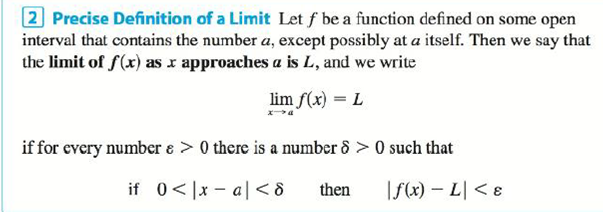Chapter 2.4, Problem 14E

Chapter
Section
Textbook Problem

Given that lim x → 2 ( 5 x − 7 ) = 3 , illustrate Definition 2 by finding values of δ that correspond to ε. = 0.1, ε = 0.05, and ε = 0.01.Definition 2To determine

To find: The number of values of δ that correspond to ε=0.1,ε=0.05 and ε=0.01.

Explanation

Given:

The limit of the function limx2(5x7) is equal to 3.

Definition used:

“Let f be a function defined on some open interval that contains the number a, except possibly at a itself. Then, the limit of f(x) as x approaches a is L, limxaf(x)=L if for every number ε>0 there is a number δ>0 such that if 0<|xa|<δ then |f(x)L|<ε”.

Calculation:

Let ε be a given positive integer. Here, f(x)=5x7, a=2 and L=5.

Consider |(5x7)8|.

|(5x7)3|=|5x73|=|5x10|=|5||x2|=5|x2|

By the definition of ε and δ, it is enough to find a number δ such that,

if 0<|x2|<δ, then |(5x7)8|<ε

Still sussing out bartleby?

Check out a sample textbook solution.

See a sample solution

The Solution to Your Study Problems

Bartleby provides explanations to thousands of textbook problems written by our experts, many with advanced degrees!

Get Started

Find the limit. 46. limni=1n3n[(1+3in)32(1+3in)]

Single Variable Calculus: Early Transcendentals, Volume I

Place the following set of n = 20 scores in a frequency distribution table.

Essentials of Statistics for The Behavioral Sciences (MindTap Course List)

Evaluate each expression: 10+42

Elementary Technical Mathematics

True or False:

Study Guide for Stewart's Multivariable Calculus, 8th

sinx=ex+ex2

Study Guide for Stewart's Single Variable Calculus: Early Transcendentals, 8th